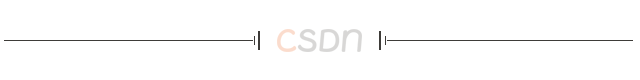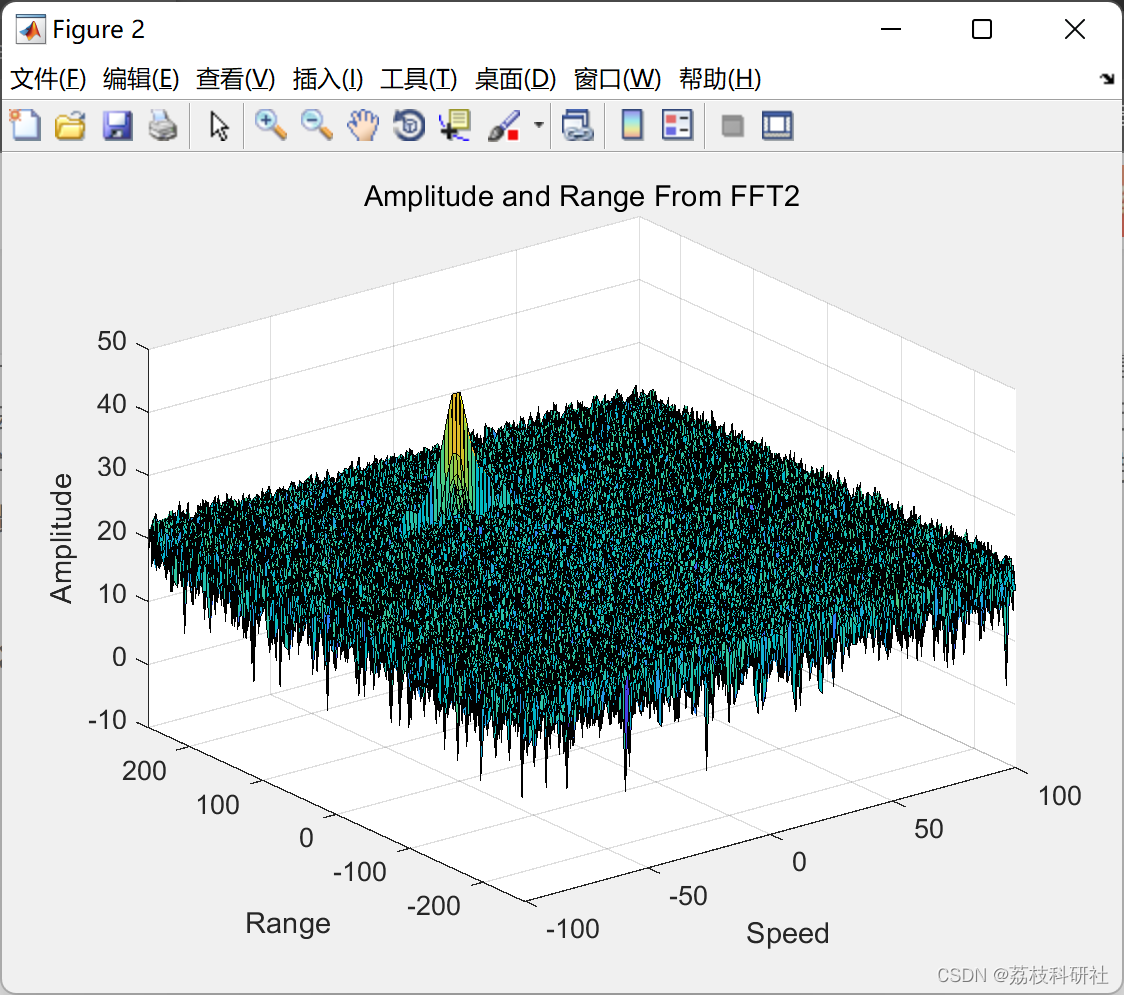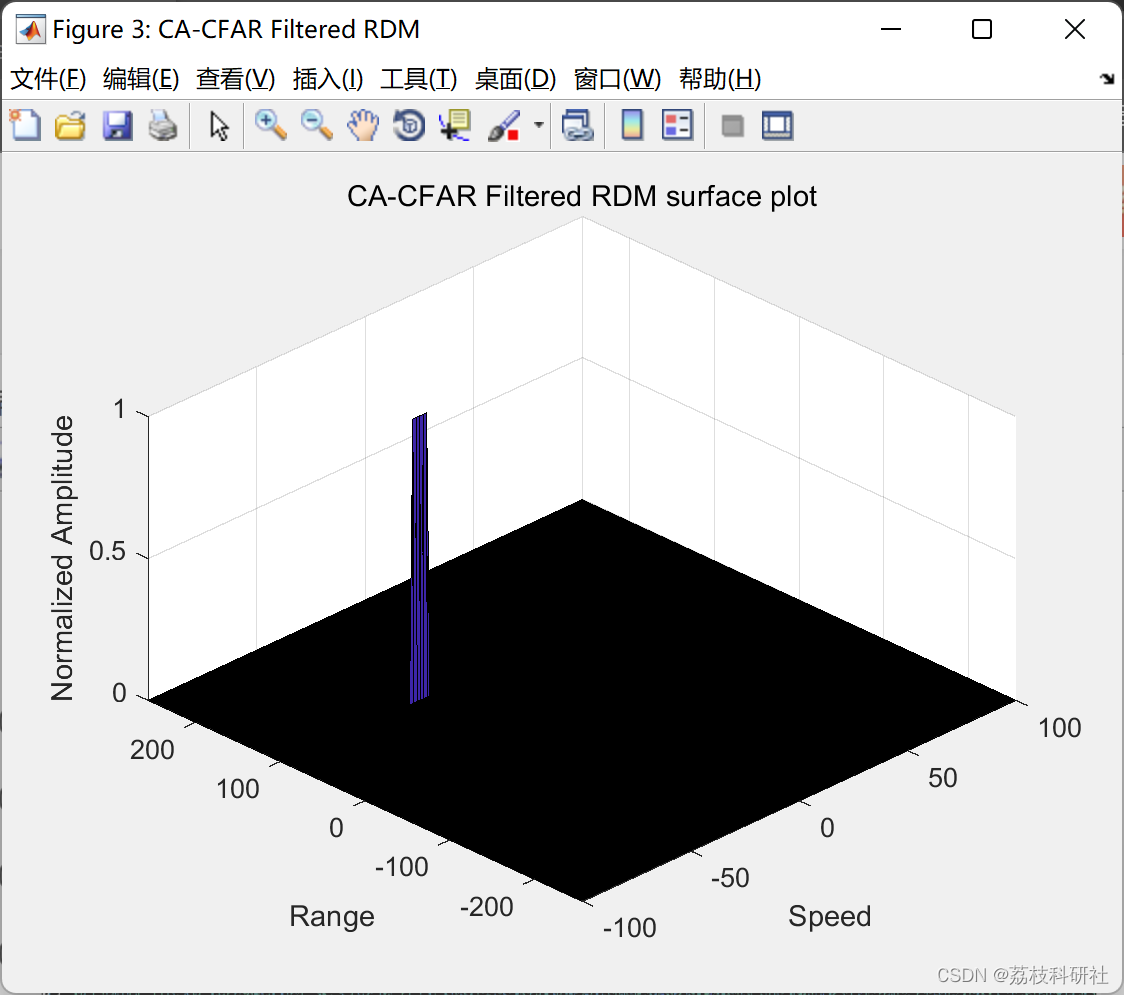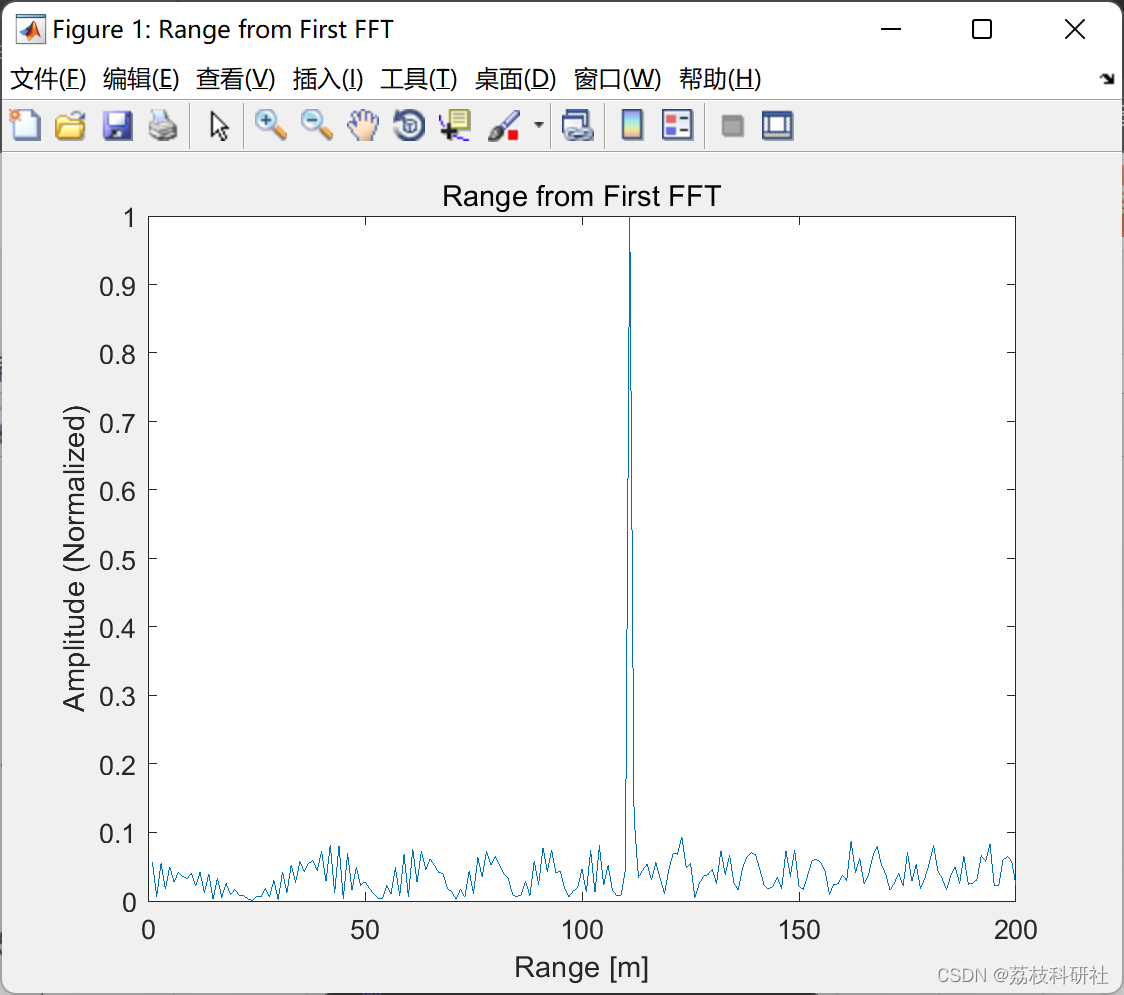# 【雷达通信】SAR雷达系统反设计及典型目标建模与仿真实现研究——目标生成与检测（Matlab代码实现）

👨‍🎓个人主页：研学社的博客

💥💥💞💞欢迎来到本博客❤️❤️💥💥

🏆博主优势：🌞🌞🌞博客内容尽量做到思维缜密，逻辑清晰，为了方便读者。

⛳️座右铭：行百里者，半于九十。

📋📋📋本文目录如下：🎁🎁🎁## 📚2 运行结果%% Signal generation and Moving Target simulation
% Running the radar scenario over the time.

for i=1:length(t)
% *%TODO*
%For each time stamp update the Range of the Target for constant velocity.
r_t(i) = range + (vel*t(i));
td(i) = (2 * r_t(i)) / c;

% *%TODO* :
%For each time sample we need update the transmitted and

Tx(i)   = cos(2*pi*(fc*t(i) + (slope*t(i)^2)/2 ) );
Rx(i)   = cos(2*pi*(fc*(t(i) -td(i)) + (slope * (t(i)-td(i))^2)/2 ) );

% *%TODO* :
%Now by mixing the Transmit and Receive generate the beat signal
%This is done by element wise matrix multiplication of Transmit and

Mix(i) = Tx(i) .* Rx(i);
end

%% RANGE MEASUREMENT

% *%TODO* :
%reshape the vector into Nr*Nd array. Nr and Nd here would also define the size of
%Range and Doppler FFT respectively.
Mix = reshape(Mix, [Nr, Nd]);

% *%TODO* :
%run the FFT on the beat signal along the range bins dimension (Nr) and
%normalize.
signal_fft = fft(Mix, Nr);

% *%TODO* :
% Take the absolute value of FFT output
signal_fft = abs(signal_fft);
signal_fft = signal_fft ./ max(signal_fft); % Normalize

% *%TODO* :
% Output of FFT is double sided signal, but we are interested in only one side of the spectrum.
% Hence we throw out half of the samples.
signal_fft = signal_fft(1 : Nr/2-1);

%plotting the range
figure ('Name','Range from First FFT')
% *%TODO* :
% plot FFT output
plot(signal_fft);
axis ([0 180 0 1]);
title('Range from First FFT');
ylabel('Amplitude (Normalized)');
xlabel('Range [m]');
axis ([0 200 0 1]);

%% RANGE DOPPLER RESPONSE
% The 2D FFT implementation is already provided here. This will run a 2DFFT
% on the mixed signal (beat signal) output and generate a range doppler
% map.You will implement CFAR on the generated RDM

% Range Doppler Map Generation.
% The output of the 2D FFT is an image that has reponse in the range and
% doppler FFT bins. So, it is important to convert the axis from bin sizes
% to range and doppler based on their Max values.

Mix=reshape(Mix,[Nr,Nd]);

% 2D FFT using the FFT size for both dimensions.
signal_fft2 = fft2(Mix,Nr,Nd);

% Taking just one side of signal from Range dimension.
signal_fft2 = signal_fft2(1:Nr/2,1:Nd);
signal_fft2 = fftshift (signal_fft2);

RDM = abs(signal_fft2);
RDM = 10*log10(RDM) ;

%use the surf function to plot the output of 2DFFT and to show axis in both
%dimensions

doppler_axis = linspace(-100,100,Nd);
range_axis = linspace(-200,200,Nr/2)*((Nr/2)/400);

figure,surf(doppler_axis,range_axis,RDM);
title('Amplitude and Range From FFT2');
xlabel('Speed');
ylabel('Range');
zlabel('Amplitude');

%% CFAR implementation

%Slide Window through the complete Range Doppler Map

% *%TODO* :
%Select the number of Training Cells in both the dimensions.
n_train_cells = 10;
n_train_bands = 8;

% *%TODO* :
%Select the number of Guard Cells in both dimensions around the Cell under
%test (CUT) for accurate estimation
n_guard_cells = 4;
n_guard_bands = 4;

% *%TODO* :
% offset the threshold by SNR value in dB
offset = 1.4;

% *%TODO* :
%Create a vector to store noise_level for each iteration on training cells
noise_level = zeros(1,1);

% *%TODO* :
%design a loop such that it slides the CUT across range doppler map by
%giving margins at the edges for Training and Guard Cells.
%For every iteration sum the signal level within all the training
%cells. To sum convert the value from logarithmic to linear using db2pow
%function. Average the summed values for all of the training%cells used. After averaging convert it back to logarithimic using pow2db.
%Further add the offset to it to determine the threshold. Next, compare the
%signal under CUT with this threshold. If the CUT level > threshold assign
%it a value of 1, else equate it to 0.

% Use RDM[x,y] as the matrix from the output of 2D FFT for implementing
% CFAR

RDM = RDM / max(RDM(:));

for row0 = n_train_cells + n_guard_cells + 1 : (Nr/2) - (n_train_cells + n_guard_cells)
for col0 = n_train_bands + n_guard_bands + 1 : (Nd) - (n_train_bands + n_guard_bands)
%Create a vector to store noise_level for each iteration on training cells
noise_level = zeros(1, 1);

for row1 = row0 - (n_train_cells + n_guard_cells) : row0 + (n_train_cells + n_guard_cells)
for col1 = col0 - (n_train_bands + n_guard_bands) : col0 + (n_train_bands + n_guard_bands)
if (abs(row0 - row1) > n_guard_cells || abs(col0 - col1) > n_guard_bands)
noise_level = noise_level + db2pow(RDM(row1, col1));
end
end
end

% Calculate threshold from noise average then add the offset
thresh = pow2db(noise_level / (2 * (n_train_bands + n_guard_bands + 1) * 2 * (n_train_cells + n_guard_cells + 1) - (n_guard_cells * n_guard_bands) - 1));
thresh = thresh + offset;

CUT = RDM(row1,col1);

if (CUT < thresh)
RDM(row0, col0) = 0;
else
RDM(row0, col0) = 1;
end

end
end

% *%TODO* :
% The process above will generate a thresholded block, which is smaller
%than the Range Doppler Map as the CUT cannot be located at the edges of
%matrix. Hence,few cells will not be thresholded. To keep the map size same
% set those values to 0.

RDM(RDM~=0 & RDM~=1) = 0;

% *%TODO* :
%display the CFAR output using the Surf function like we did for Range
%Doppler Response output.
figure('Name', 'CA-CFAR Filtered RDM')
surf(doppler_axis, range_axis, RDM);
%colorbar;
title( 'CA-CFAR Filtered RDM surface plot');
xlabel('Speed');
ylabel('Range');
zlabel('Normalized Amplitude');
view(315, 45);

## 🎉4 参考文献

易重辉. 基于深度学习的低空监视雷达目标检测的研究[D].四川大学,2021.DOI:10.27342/d.cnki.gscdu.2021.000528.

09-21460
11-23209
09-07841
05-222321
12-0498
11-27420
01-118244
03-20800
12-03795
09-072168
03-18577

### “相关推荐”对你有帮助么？

•非常没帮助
•没帮助
•一般
•有帮助
•非常有帮助被折叠的  条评论 为什么被折叠?到【灌水乐园】发言¥2 ¥4 ¥6 ¥10 ¥20余额支付 (余额：-- )扫码支付获取中扫码支付点击重新获取扫码支付1.余额是钱包充值的虚拟货币，按照1:1的比例进行支付金额的抵扣。
2.余额无法直接购买下载，可以购买VIP、C币套餐、付费专栏及课程。余额充值